Евразийский
научный
журнал

Срочная публикация научной статьи

+7 995 770 98 40
+7 995 202 54 42
info@journalpro.ru# Application of the fuzzy linear programming in the proportions of problem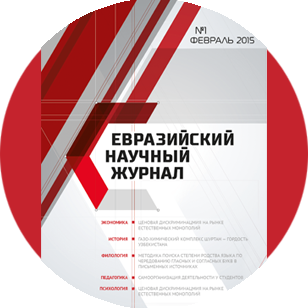Поделитесь статьей с друзьями:
Автор(ы): Ons Edin Mousa
Рубрика: Физико-математические науки
Журнал: «Евразийский Научный Журнал №3 2016»  (март)
Количество просмотров статьи: 3145
Application of the fuzzy linear programming in the proportions of problem
Ons Edin Mousa Al-Mustansiriya University

Modeling and optimization under a fuzzy environment are called fuzzy modeling and fuzzy optimization. Fuzzy multi-objective linear programming is one of the most frequently applied in fuzzy decision making techniques. Although, it has been investigated and expanded for more than decades by many researchers and from the varies point of view, it is still useful to develop new approaches in order to better fit the real world problems within framework of fuzzy multi-objective linear programming.

However, when formulating the multi-objective programming  problem which closely describes and represents the real decision situation, various factors of the real system should be reflected in the description of the objective functions and the constraints. Naturally, these objective functions and the constraints involve many parameters whose possible values may be assigned by the experts. In the traditional approaches, such parameters are fixed at some values in an experimental or subjective manner through the expert's understanding of the nature of the parameters. Unfortunately, real world situations are often not deterministic. There exist various types of uncertainties in social, industrial and economic systems, such as randomness of occurrence of events, imprecision and ambiguity of system data and linguistic vagueness, etc. which come from many ways, including errors of measurement, deficiency in history and statistical data, insufficient theory, incomplete knowledge expression and the subjectivity and preference of human judgment, etc. As pointed out by Zimmermann (1978), various kinds of uncertainties can be categorized as stochastic uncertainty and fuzziness.

Stochastic uncertainty relates to the uncertainty of occurrences of phenomena or events. Its characteristics, lie in that descriptions of information are crisp and well defined; however, they vary in their frequency of occurrence. The systems with this type of uncertainty are called stochastic systems, which can be solved by stochastic optimization techniques using probability theory.

In some other situations, the decision-maker does not think about the frequently used probability distribution which is always appropriate, especially when the information is vague. It may be related to human

language and behavior, imprecise/ ambiguous system data. Such types of uncertainty are called fuzziness. It cannot be formulated and solved effectively by traditional mathematics-based optimization techniques and probability based stochastic optimization approaches.

Multi-objective Linear Programming (MOLP) Problem:

Multi-objective Linear Programming (MOLP) Problems is an interest area of research, since most real-life problems have a set of conflict objectives. A mathematical model of the MOLP problem can be written as follows: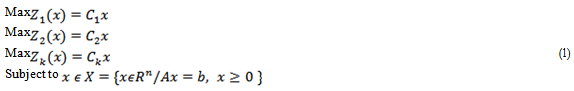where   x  is an   n – dimensional vector of decision variables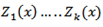are k  – distinct linear objective function of the decision vector . A is an mxn constraint matrix, b is an m – dimensional constant vector.

Definition 3. 1. (Complete Optimal Solution) The point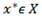is said to be a complete optimal solution of the MOLP problem (1), if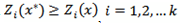for all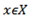In general, when the objective functions conflict with one another, a complete optimal solution may not exist and hence, a new concept of optimality, called Pareto optimality, is considered.

Definition 3. 2. (Pareto Optimal Solution) The point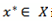is said to be a Pareto optimal solution if there does not exist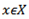such that if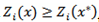for all i and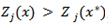for at least one j

Fuzzy Multi-objective Linear Programming (FMOLP) Problem:

The model (1), all coefficients of A, b and C are crisp numbers. However, in the real-world decision

problems, a decision maker does not always know the exact values of the coefficients taking part in the problem, and that vagueness in the coefficients may not be a probabilistic
type. In this situation, the decision maker can
model inexactness by means of fuzzy parameter. In
this section we consider a FMOLP problem with fuzzy technological coefficients and fuzzy resources. A mathematical model of the FMOLP problem can be written as
follows: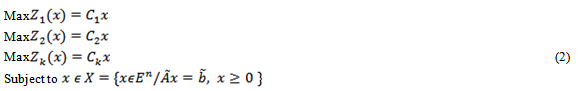where x is an n – dimensional vector of decision variables.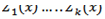are k - distinct linear objective

function of the decision vector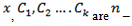dimensional cost factor vectors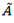is an mxn constraint fuzzy matrix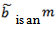- dimensional constant fuzzy vector (fuzzy resources).

The membership
function of the fuzzy matrix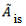: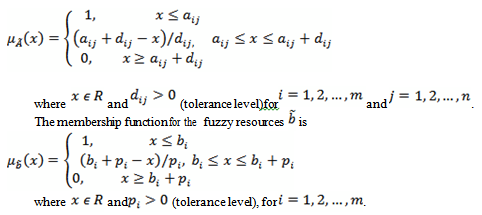Solution Methodology and Algorithm:

In this section, we first fuzzify the objective function in order to defuzzificate the problem (2). It is done by

calculating the lower and upper bounds of the optimal values. The bounds of the optimal values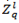and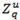are obtained by solving the standard linear programming problems.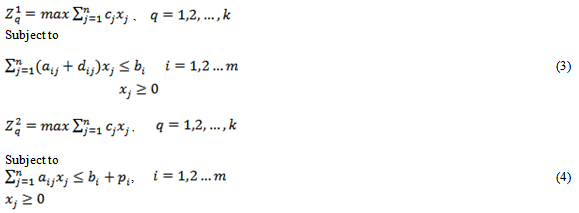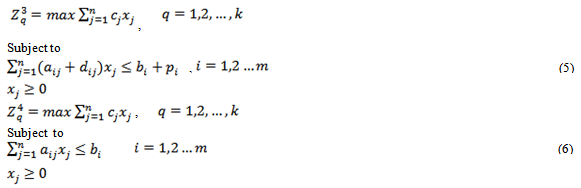Let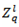= min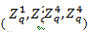and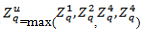the objective function takes the values between and while the technological coefficients take values between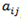and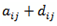and the right hand side numbers takes the value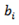and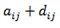then the fuzzy set of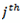timal value,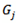which sub set for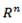is defined by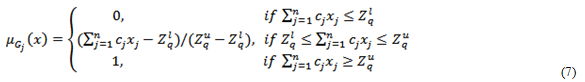The fuzzy set of the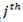constraint,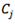which subset for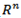, is defined by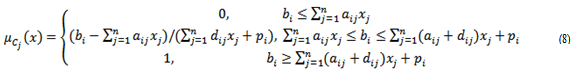By using the definition of the fuzzy decision proposed by Bellman and Zadeh, we have: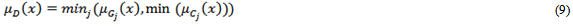In this case the optimal fuzzy decision is a solution of the problem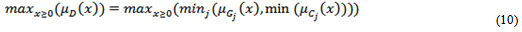Consequently, the problem (2) is reduced to the following optimization problem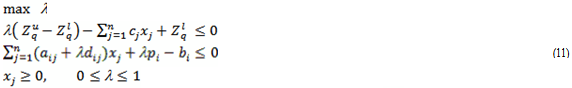Notice that, the problem (11) containing the cross product terms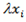are  not convex Therefore, the solution of the problem requires the special approach adopted for solving general non-convex optimization problems.

The Algorithm of the Fuzzy Decisive Set Method:

This method is based on the idea that, for a fixed value of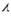, the problem (11) is converted in to linear

programming problem. Obtaining the optimal solution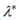is equivalent to determining the maximum value of so that the feasible set is nonempty. The algorithm of this method for the problem (11) is presented below.

Algorithm:

Step 1:

Set λ =1 and test whether a feasible set satisfying the constraints of the problem (11) exists or not using

phase one of the simplex method. If a feasible set exists, set λ = 1  . Otherwise, set λ = u  and and λ = 1 go to the next step.

Step 2:

For the value of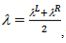update the value of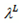and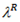using the bisection method as follows: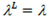, if feasible set is nonempty for λ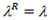, if feasible set is empty for λ

Consequently, for each λ, test whether a feasible set of the problem (11) exists or not using phase one of the Simplex method and determine the maximum value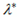satisfying the constraints of the problem (11)

Numerical Example:

Consider the following FMOLPP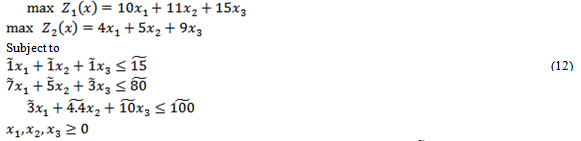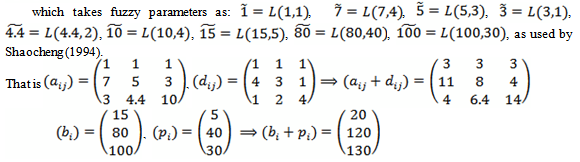For defuzzification of the problem (12), we first fuzzify the objective function. This is done by calculating the lower and upper bounds of the optimal values first. The bounds of the optimal values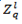and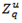are obtained by solving the standard linear programming problems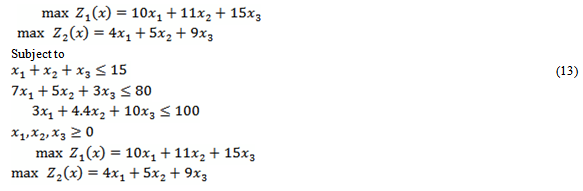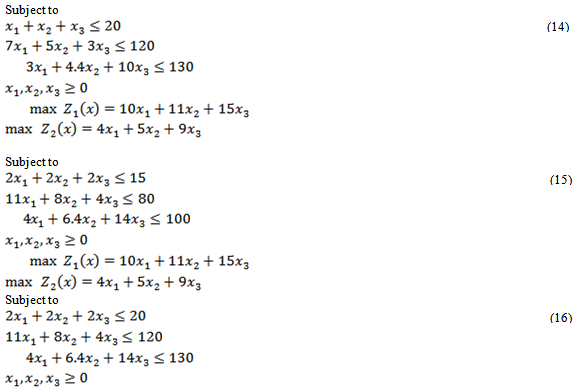Optimal values of these problems are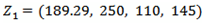and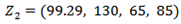respectively.
Therefore,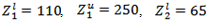and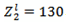.By using these optimal values, the problem (12) can be reduced by the following non-linear programmig problem: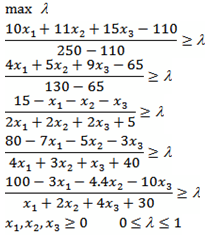that is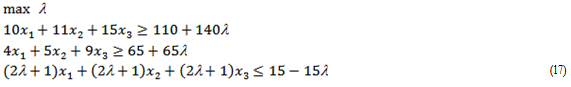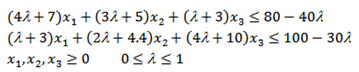Let us solve the problem (17) by using fuzzy decisive set method.

For λ = 1, the problem can be written as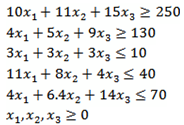Since the feasible set is empty, by taking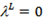and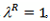, the new value of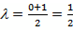is tried

For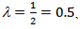the problem (17) can be written as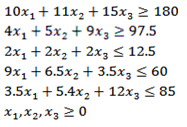Since the feasible set is empty, by taking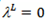and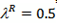, the new value of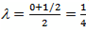For λ = 0.25, the problem (17) can be written as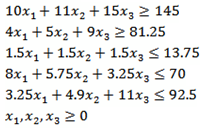Since the feasible set is empty, by taking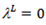and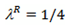the new value of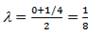is tried

For λ = 0.125 , the problem (17) can be written as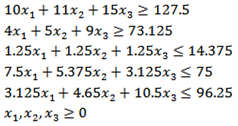Since the feasible set is nonempty, by taking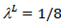and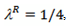the new value of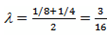is tried

For λ = 0.19 , the problem (17) can be written as